# Deep Learning Interview Questions Data Science

Prepare better with the best interview questions and answers, and walk away with top interview tips. These interview questions and answers will boost your core interview skills and help you perform better. Be smarter with every interview.

• 4.6 Rating
• 61 Question(s)
• 70 Mins of Read

## Beginner

Deep learning is large neural networks. It is a machine learning technique that teaches the computers to do what comes naturally to humans, learn by example. It is a key technology behind driverless cars, also deployed in medical research to detect the cancer cells which may as well achieve state of the art accuracy sometimes exceeding the human level performance.

The term “deep” usually refers to the number of hidden layers in the neural network. These models are trained by using large sets of labeled data and neural network architectures that learn features directly from the data without the need for manual feature extraction. Some of the deep neural networks are MLP, CNNs

• Google and Facebook are translating text into hundreds of languages at a time. This is being done through some deep learning models being applied to NLP tasks and is a major success story.
• Conversational agents like Siri, Alexa, Cortana basically work on simplifying the speech recognition techniques through LSTMs and RNNs.
• Deep learning is being used in impactful computer vision applications such as OCR (Optical Character Recognition) and real-time language translation
• Multimedia sharing apps like Snapchat and Instagram apply facial feature detection which is another application of deep learning.
• Deep Learning is being used in the Healthcare domain to locate malignant cells and other foreign bodies in order to detect complex diseases.
• sales forecasting
• industrial process control
• customer research
• data validation
• risk management
• target marketing

A perceptron (a single neuron model) is always feedforward, that is, all the arrows are going in the direction of the output. In addition, it is assumed that in a perceptron, all the arrows are going from layer ‘i’ to layer ‘i+1’, and it is also usual (to start with having) that all the arcs from layer ‘i’ to ‘i+1’ are present.

Finally, having multiple layers means more than two layers, that is, you have hidden layers. It consists of 3 layers of nodes: a. Input, b. Hidden c. and output.

A perceptron is a network with two layers, one input and one output whereas a multilayered network means that you have at least one hidden layer (we call all the layers between the input and output layers hidden).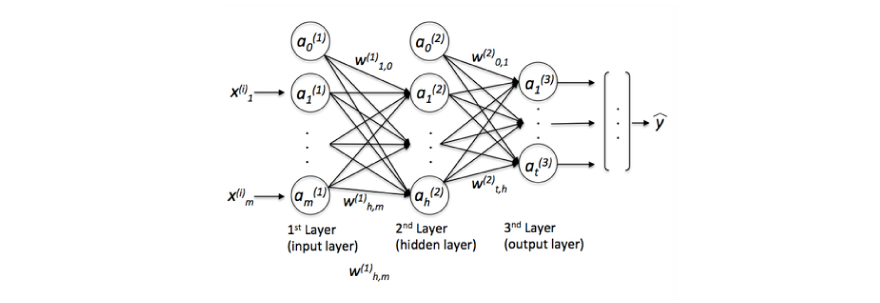(where y_hat is the final class label that we return as the prediction based on the input x, ‘a’ is the activated neurons and ‘w’ are the weight coefficients.)

Input layers are the training observations that are fed through the neurons.

It computes a linear function (z = Wx+b) followed by an activation function.

These are the intermediate layers between input and output which help the neural networks to learn the complicated relationships involved in data.

The sigmoid function is a logistic function bounded by 0 and. This is an activation function that is continuous and differentiable. It is nonlinear in nature that helps to increase the performance making sure that small changes in the weights and bias causes small changes in the output of the neuron.where Alpha is the slope parameter of the above function.

Maxout function has been found by Ian Goodfellow, a research scientist at Google brain in 2013. It facilitates optimization by dropout and improves the accuracy of dropout’s fast approximate model averaging technique. It learns not just the relationship between the hidden units, but also the activation function of each hidden unit.

• It can be trained as a supervised learning problem
• It is applicable when the input/output is a sequence. (i.e. a sequence of words)

As a result, the weights are then pushed towards becoming smaller (closer to 0)

Normalizing the input x makes the count function faster to optimize.

• We should try using adam optimizer
• Try for better random initialization for the weights
• Try tuning the learning rate ‘alpha’
• Must as well try to initialize the weight to 0.

We use epsilon during normalization to avoid the division by zero.

We must try random values, rather than carrying out systematic research because we don’t know which hyperparameter is more important than other. At the same time, the choice of alpha (learning rate) also matters a lot.

We use it to pass the variables computed during the forward propagation to the corresponding backward propagation step. It contains useful values for backward propagation to compute the derivatives.

Data normalization is very important preprocessing step, used to rescale values to fit in a specific range to assure better convergence during backpropagation. In general, it boils down to subtracting the mean of each data point, dividing by its standard deviation.

Neural network converts data in such a form that it would be better to solve the desired problem and hence, called representation learning.

Logistic regression has a non-linear activation function that squashes the linear input meaning that it returns the conditional probability, but the weight coefficients of this model are essentially a linear combination. Hence, a generalized linear model.

Neural networks make use of ReLU activation functions, having the power to approximate the non-linear function.

The goal of an activation function is to introduce nonlinearity/a non-linear decision boundary via non-linear combinations of the weighted inputs into the neural network so that it can learn more complex function i.e. converts the processed input into an output called the activation value. Without it, the neural network would be only able to learn function which is a linear combination of its input data.

Some of the examples are – Step Function, ReLU, Tanh, Softmax.

1.  It is a measure to evaluate how good your model's performance is. Also referred to as ‘ Loss/Error’
2. It is used to compute the error of the output layer during back propagation.
3. Mean squared error or Sum of squared errors is an example of the popular cost function.

C = ½ (y – y_hat)^2

Where c = cost function, y = Original output & y_hat = Predicted output.

Gradient descent is an optimization algorithm to minimize the cost function in order to maximize the performance of the model. It aims to find the local or the global minima of the function. So, if your cost is a function of K variables, then the gradient is the length-K vector that defines the direction in which the cost is increasing most rapidly and you follow the negative of the gradient to the point where the cost is a minimum.

Backpropagation is a method to update the weights in the neural network by taking into account the actual output and the desired output. It is a training algorithm used for a multilayer neural network. It moves the error information from the end of the network to all the weights inside the network and thus allows for efficient computation of the gradient. (The technique used to minimize the cost function is called “gradient descent”.)1.  Forward propagation of the training data through the network in order to generate the output.
2.  Compute the error derivative using the target value and the output value with respect to output activations.
3.  Then, we backpropagate to compute the derivative of the error with respect to the output activations in the previous layer.
4.  We continue the same for all the hidden layers.
5.  Use the previously calculated derivatives for output and all the hidden layers, to calculate the derivative of the error w.r.t weights.
6.  Update the weights.In feed forward neural networks –

1. The signal travels in one direction, from input to the output.

2. There are No feedbacks or loops

3. It considers only the current input

4. It cannot memorize the previous inputs. (eg. CNNs)

In Recurrent neural networks –

1. The signal travels in both the directions i.e. they can pass the information onto themselves.

2. It considers the current input along with the previously received inputs to generate the output of a layer.

• One to Many

This is the simple network with one input and multiple outputs. Example: It helps you caption an image, where the picture goes through the CNN model and then fed to the RNN.

• Many to One

Example: It can be used in sentiment analysis and text mining, where you have a lot of text such as a customer’s comment and you need to gauge that what’s the chance that this comment is positive or negative.

• Many to Many

Translations such as Google translator and generating subtitles for the movies is an example of many to many types of network.

• Softmax Function-

Softmax function calculates the probabilities distribution of the event over ‘n’ different events.In general way of saying, this function will calculate the probabilities of each target class over all possible target classes. Later the calculated probabilities will be helpful for determining the target class for the given inputs.

So, softmax function squashes the outputs of each unit to be between 0 and 1, just like a sigmoid function. But it also divides each output such that the total sum of the outputs is equal to 1 (check it on the figure above) where an output is equivalent to a categorical probability distribution.

Mathematically the softmax function is shown to the right, where z is a vector of the inputs to the output layer (if you have 10 output units, then there are 10 elements in z). And again, j indexes the output units, so j = 1, 2, ..., K.• ReLU –

Also, known as Rectified linear units. It reproduces output 0 if the input is less than 0, and raw output otherwise. That is, if the input is greater than 0, the output is equal to the input.

It results in much faster training for larger networks.

Hyperparams can’t learn from the data, they are set before the training phase such as –

1. A number of epochs – In the context of training the model, epoch is a term used to refer to one iteration where model sees the whole training set to update its-weights.
1. Batch Size – refers to the number of training examples in one forward/backward pass.
1. Learning rate – The learning rate, often noted as ‘alpha’, indicates at which pace the weight gets updated. It can be fixed or adaptively changed. The current most popular method is called ‘Adam’, a method that adapts the learning rate.

If the learning rate too high:

1. This may cause an undesirable divergent behavior to the loss function due to the drastic updates in weights.
2. It may as well fail to converge or even diverge

If the learning rate too low:

1. This will lead to very slow progress in training of the model as we make tiny updates in weights.
2. It’ll take many updates before reaching a minimum point.

Dropout is a regularization technique for reducing overfitting in neural networks by dropping out the neurons with probability p>0. It forces the model to avoid relying too much on particular sets of features.

It is a step of hyperparams, which normalizes the batch. To facilitate learning, we typically normalize the initial values of our parameters by initializing them with zero mean and unit variance. As training progresses and we update parameters to different extents, we lose this normalization, which slows down training and amplifies changes as the network becomes deeper.

Batch normalization re-establishes these normalizations for every mini-batch and changes are back-propagated through the operation as well. By making normalization part of the model architecture, we are able to use higher learning rates and pay less attention to the initialization parameters. Batch normalization additionally acts as a regularizer, reducing/ even eliminating the need for Dropout.

It is usually done after a fully connected/convolutional layer and before a non-linearity layer which aims at allowing higher learning rates and reducing the strong dependence on initialization.

1. Batch Gradient descent – Vanilla gradient descent, aka batch gradient descent, calculates the gradient of the whole dataset and perform just one update at each iteration. Batch gradient descent is guaranteed to converge to the global minimum for convex error surfaces and to a local minimum for non-convex surfaces.
1. Stochastic gradient descent – It uses only a single training example ( each training example ) to calculate the gradient and update the parameters. It is fast and performs frequent updates with a high variance.

It has been shown that when we slowly decrease the learning rate, SGD shows the same convergence behavior as batch gradient descent, almost certainly converging to a local or the global minimum for non-convex and convex optimization respectively.

Overfitting happens when a model learns the details as well as the noise in the training data to the degree that it adversely impacts the execution to the model on new information.

It is more likely to occur with non-linear models that have more flexibility when learning a target function.

Underfitting, it is when a model is neither trained well on the training dataset nor it can generalize the new information properly. It usually happens when there is less and improper data to train a model.

And results in poor performance and accuracy.

Combating overfitting and underfitting:

• K-fold cross-validation - Resample the data to estimate the accuracy.
• Having a validation dataset to evaluate the model.
• Regularization techniques such as Weight regularization ( Lasso, ridge), Dropout regularization, early stopping (It is when you stop the training process  as soon as the validation loss reaches a plateau or starts to increase)
• Initializing all the weights to zero –

When you set all the weights to 0, the derivative w.r.t loss function is the same for every ‘w’ in W^l, thus all the weights have the same values in a subsequent iteration. And thus setting It to zero, makes the model no better than the linear model. (equivalent to linear model)

`W = np.zeros(layer_size[l]. layer_size[l-1]) `
• Initializing weights Randomly –

Here, weights are assigned randomly by initializing them very close to zero.

`np.random.randn(size_l, size_l-1) `

It gives better accuracy to the model since every neuron performs different computations. But this can potentially lead to 2 issues – Vanishing gradient and Exploding gradient.

The problem of the vanishing gradient was first discovered by Sepp (Joseph) Hochreiterback in 1991.

1. Now that we know, information travels through time in RNNs, which means that information from previous time points is used as input for the next time points. Secondly, you can calculate the cost function, or your error, at each time point.
2. Basically, during the training, your cost function compares your outcomes to your desired output.
3. You’ve calculated the cost function, and now you want to propagate your cost function back through the network because you need to update the weights, meaning that you’ve to propagate all the way back through the time to these neurons.
4. This is where the problem lies, for any activation function, abs(dW) will get smaller and smaller as we go back with every layer during back propagation.

Since the weight update is minor and results in slower convergence. This makes the optimization of the loss function slow. In the worst case, this may completely stop the neural network from training further or may as well return half of the network not trained.

As a result of setting weights in the network to zero, all the neurons at each layer are producing the same output and the same gradients during backpropagation.
The network can’t learn at all because there is no source of asymmetry between neurons. That is why we need to add randomness to the weight initialization process.

Convolutional Neural Networks are very similar to ordinary Neural Networks. They are made up of neurons that have learnable weights and biases. Each neuron receives some inputs, performs a dot product and optionally follows it with a non-linearity. The whole network still expresses a single differentiable score function: from the raw image pixels on one end to class scores at the other. And they also have a loss function (e.g. SVM/Softmax) on the last (fully-connected) layer.

The change is that ConvNet architectures make the explicit assumption that the inputs are images, which allows us to encode certain properties into the architecture. These then make the forward function more efficient to implement and vastly reduce the number of parameters in the network.1. Convolutional Layer – A layer that performs the convolutional operation on 2 functions to produce a third function that expresses how the shape of the one is modified by the another.
2. ReLU Layer – It brings non-linearity to the network and converts all the negative pixels to the zero, where your output is a rectified feature map.
3. Pooling layer – A down sampling operation that reduces the dimensionality of the feature map.

As mentioned above, it is a down-sampling operation that is typically applied after a convolutional layer, which does some sort of spatial invariance, to reduce the spatial dimensions of the CNN.

It creates a pooled feature map sliding a filter matrix over the input matrix. In particular, max and average pooling are special kinds of pooling where max and average values are taken, respectively.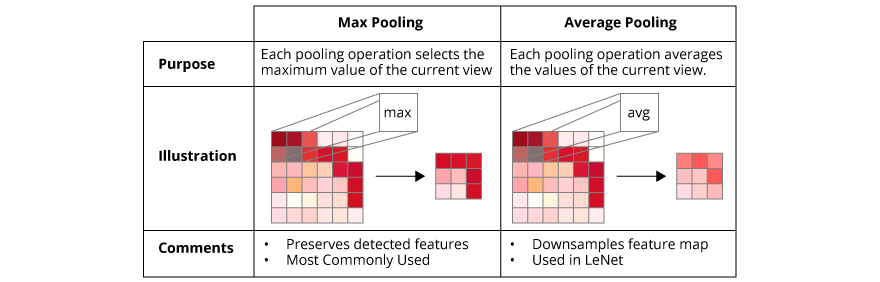Source

The fully connected layer operates on the flattened input where each input is connected to all the neurons. If present, the fully connected layers are usually found towards the end of the CNN architecture and can be used to optimize the objectives such as class scores.

It is used to define a loss function in machine learning and optimization. Also called the log loss, measures the performance of the classification model whose output is a probability value between 0 and 1.

It is a regularization technique that stops the training process as soon as the validation loss reaches a plateau or starts to increase.

In the case of the exploding gradient, you can:

• Truncated Backpropagation - Stop back-propagating after a certain point, which is usually not optimal because not all the weights get updated.
• Penalties - Penalize or artificially reduce the gradient
• Gradient clipping - Put a maximum limit on a gradient

In case of vanishing gradient:

• Weight initialization - Initialize the weight so that the potential of the vanishing gradient is minimized
• Have Echo State Networks
• Have Long Short-Term Memory Networks (LSTMs)

LSTMs, are special kind of Recurrent Neural Networks that are capable of learning long-term dependencies i.e. remembering the information for a longer period of time is their default behavior.

There are 3 steps in the process:

1. Decides what to forget and what to remember
2. Selectively updates the cell state values
3. Decides what part of the current state make it to the output
• A gated recurrent unit (GRU) is basically an LSTM without an output gate and has 2 gates – reset and update gates, which therefore fully writes the contents from its memory cell to the larger net at each time step.
• The GRU unit controls the flow of information like the LSTM unit, but without having to use a memory unit. It just exposes the full hidden content without any control.
• GRU is relatively new, and from my perspective, the performance is on par with LSTM, but computationally more efficient (less complex structure as pointed out). So we are seeing it being used more and more.

TF-IDF, which stands for term frequency-inverse document frequency, is a scoring measure widely used in text summarization. TF-IDF is intended to reflect how relevant a term is in a given document.

• The intuition behind it is that if a word occurs multiple times in a document, we should boost its relevance as it should be more meaningful than other words that appear fewer times (TF).
• At the same time, if a word occurs many times in a document but also along with many other documents, maybe it is because this word is just a frequent word; not because it was relevant or meaningful (IDF).

For example: Consider a document containing 100 words wherein the word Rajat appears 3 times.

1. The term frequency (tf) for ‘Rajat’ is then TF = (3 / 100) = 0.03.
2. Now, assume we have 10 million documents and the word ‘Rajat’ appears in 1000 of these. Then, the inverse document frequency (idf) is calculated as IDF = log(10,000,000 / 1,000) = 4.
3. Thus, the Tf-idf weight is the product of these quantities TF-IDF = 0.03 * 4 = 0.12.

Higher dropout rate says that more neurons are active. So there would be less regularization.

It is the ability to approximate any given function. The higher model capacity is the larger amount of information that can be stored in the network.

VGG is a convolutional neural network architecture named after the Visual Geometry Group from Oxford, who developed it.

Built using:

• Convolutions layers (used only 3*3 size )
• Max pooling layers (used only 2*2 size)
• Fully connected layers at the end

Application:

• Given an image, it finds object name in the image
• It can detect any one of 1000 images
• It takes input image of size 224 * 224 * 3 (RGB image)

For code snippets to extract features with VGG: https://keras.io/applications/

This line is used to convey the fact that we wish to tune the value of the keep probability of Dropout and find the best fit among the range of real numbers between 0 and 1.Data compression is a big topic that’s used in computer vision, computer networks, computer architecture, and many other fields. The point of data compression is to convert our input into a smaller representation that we recreate, to a degree of quality. This smaller representation is what would be passed around, and, when anyone needed the original, they would reconstruct it from the smaller representation.

Autoencoders are unsupervised neural networks that use machine learning to do this compression for us. The aim of an autoencoder is to learn a compressed, distributed representation for the given data, typically for the purpose of dimensionality reduction.

Steps include –

1. Let’s take a neural network that has 3 layers. (refer to the image attached)
2. The network is then trained to reconstruct its inputs. Here, input neurons are equal to the output neurons.
3. And, the network’s target output is same as the input. It uses the dimensionality reduction to restructure the input.

It works by compressing the input to a latent-space representation and then reconstructing the output from the representation.

operator indicates the element-wise multiplication. Element-wise multiplication requires the same dimension between two matrices. It's going to be an error.

C = a + b.T

```for(i in range(1, len(layer_dims))):
parameter[‘W’ + str(i)] = np.random.randn(layers[i], layers[i - 1])) * 0.01
parameter[‘b’ + str(i)] = np.random.randn(layers[i], 1) * 0.01```

These are based on the idea that the output at a time may not only depend on the previous elements in the sequence. But also future elements. For example:

To predict a missing word in a sequence you want to look at both the left and the right context. Bidirectional RNNs are quite simple. They are just two RNNs stacked on top of each other. The output computes based on the hidden state of both RNNs.

A regularization technique (such as L2 regularization) that results in gradient descent shrinking the weights on every iteration.

LSTMs have a chain like structure similar to standard RNNs. But instead of having a single neural network layer, there are four, interacting in a very special way.

In the below figure,

1. Ct-1: is the input from memory cell in time point t;
2. Xt: input in time point t;
3. ht: output in time point t that goes to both the layers and hidden layer in the next time point.

Thus, every block has three inputs (xt, ht-1, and ct-1) and two outputs (ht and ct).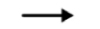denotes vector transfer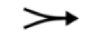Line merging here denotes concatenation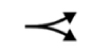shows that the information is copied and further transferred in 2 different directions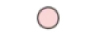points to the pointwise operations like vector addition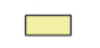this yellow box is termed as a learned neural network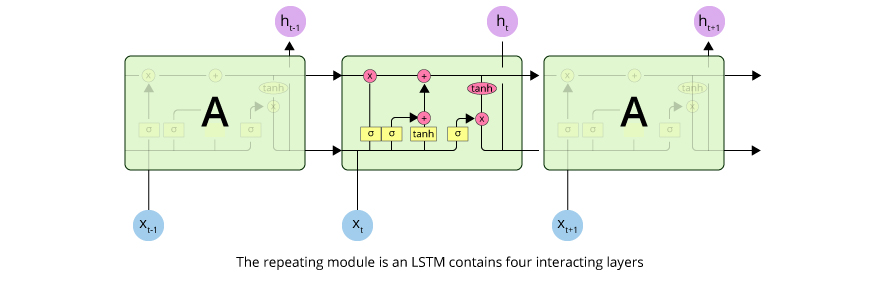Source

Understanding Step-by-Step: Idea behind the LSTMs

1. We’ve new value xt and value from the previous node ht-1.
2. In the first step, these values are combined together to go through the sigmoid activation function, where it is decided if the forget valve should be open, closed or open to some extent.
3. In the next step, the same values, or actual vectors of values, go in parallel through layer operation tanh, where we decide what value we’re going to pass to the memory pipeline, and another layer of operation ‘sigmoid’, where it is decided, if that value is going to be passed to the memory pipeline and to what extent.
4. Then, we have a memory flowing through the top pipeline. If we have forget the valve open and memory valve closed then the memory will not change. Otherwise, if we have forget valve closed and memory valve open, the memory will be updated completely.
5. Finally, we’ve got xt and ht-1 combined to decide what part of the memory pipeline is going to become the output of this module.

The LSTM does have the ability to remove or add information to the cell state, carefully regulated by structures called gates. Gates are a way to optionally let information through. They are composed out of a sigmoid neural net layer and a pointwise multiplication operation.

An LSTM has three of these gates, to protect and control the cell state.

• The first step in our LSTM is to decide what information we’re going to throw away from the cell state. This decision is made by a sigmoid layer called the forget gate layer. It looks at ht−1and xt, and outputs a number between 0 and 1 for each number in the cell state Ct−1.

Where 1 represents- completely keep this while,0 represents- completely get rid of this.

• The next step is to decide what new information we’re going to store in the cell state. This has two parts. First, a sigmoid layer called the input gate layer decides which values we’ll update. Next, a tanh layer creates a vector of new candidate values, ~Ct, that could be added to the state.
• Finally, we need to decide what we’re going to output. This output layer will be based on our cell state, but will be a filtered version. First, we run a sigmoid layer which decides what parts of the cell state we’re going to output. Then, we put the cell state through tanh (to push the values to be between −1 and 1) and multiply it by the output of the sigmoid gate, so that we only output the parts we decided to.

For further clarification, please refer: http://colah.github.io/posts/2015-08-Understanding-LSTMs/

Here are few ideas to keep in mind when manually optimizing the hyperparameters:

• Using regularization methods that include l1, l2, dropout, and others help avoid overfitting.
• More data is always better
• Train over multiple epochs
• Early stopping - evaluate the test set performance at each epoch to know when to stop
• Learning rate is the single most important parameter
• For LSTMs use soft sign over tanh as it is faster and less prone to saturation (~0 gradients)
• Optimizers such as RMSprop, AdaGrad or momentum are usually good choices
• Finally remember data normalization, MSE Loss function + identity activation function for regression, Xavier weight initialization

A Boltzmann machine is a network of symmetrically coupled stochastic binary units. In other words, they are shallow 2 layer neural nets that make stochastic decisions whether a neuron should be on or off where 1st layers is the visible layer and the 2nd layer is the hidden layer.

In a Boltzmann machine, nodes are connected to each other across the layers but no two nodes of the same layer are connected. Hence, also known as Restricted Boltzmann Machine.Fig: Boltzmann machine

## Description

Prepare better with the best interview questions and answers, and walk away with top interview tips. These interview questions and answers will boost your core interview skills and help you perform better. Be smarter with every interview.